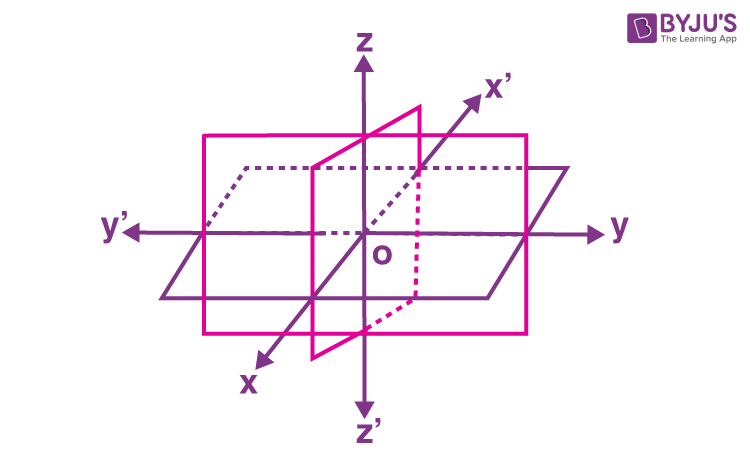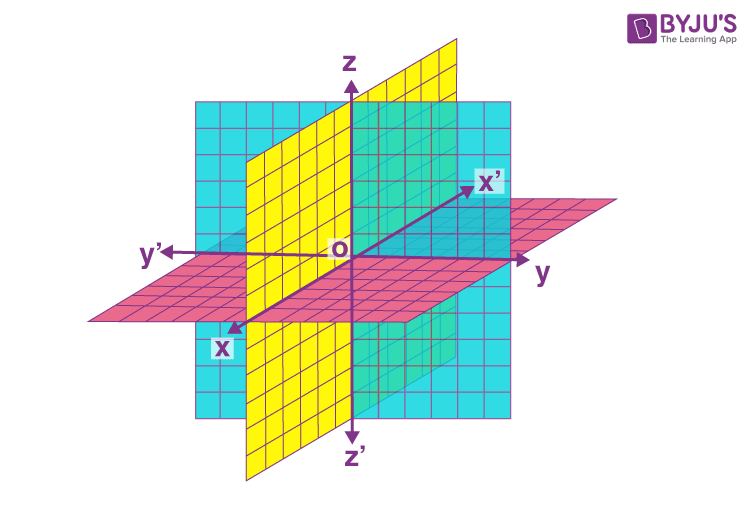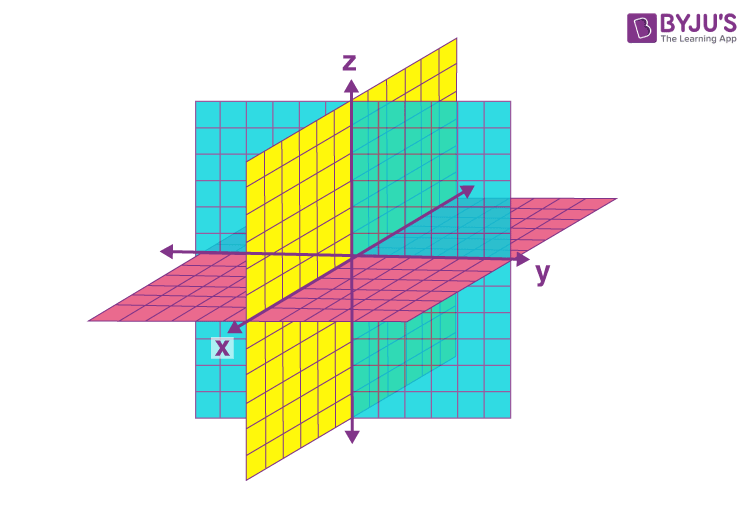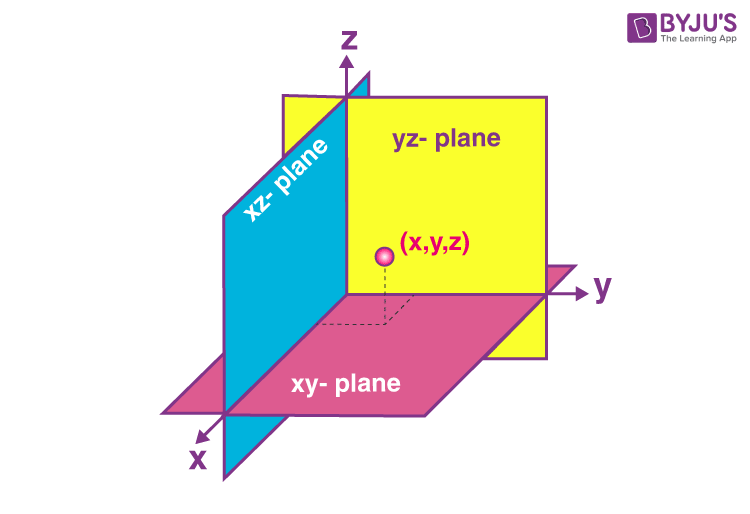# Coordinate Axes and Coordinate Planes in Three Dimensional Space

A three dimensional space is the set of all such locations in n-dimensional space when n = 3. It is commonly represented by the symbol ℝ3. In other words, three-dimensional space or 3D space, or, tri-dimensional space, is a geometric context in which three values called parameters are required to determine the position of a point. These parameters are called coordinates of a point in three dimensions.

The below figure shows the coordinate axes and planes in three dimensions.## What are Coordinate Axes in Three Dimensional Space?

Suppose three planes intersect at a point O such that these three planes are mutually perpendicular to each other, as shown in the below figure.These three planes intersect along the lines X′OX, Y′OY and Z′OZ, and are respectively called the x, y and z-axes. However, point O is called the origin of the coordinate system. The representation of coordinate axes in three dimensions is given below.As these lines are mutually perpendicular to each other, they constitute the rectangular coordinate system.

## What are Coordinate Planes in Three Dimensional Space?

The planes XOY, YOZ and ZOX, are called the XY-plane, YZ-plane, and the ZX-plane, respectively; these are also known as the three coordinate planes. These can also be denoted using small letters such as xy-plane, yz-plane, and zx-plane along the x, y and z-axes. The below figure depicts the coordinate planes of a three-dimensional space.The positive and negative axes can be determined as explained below:

• The distances measured from the XY-plane upwards in the direction of OZ are considered positive, and those marked downwards in the direction of OZ′ are considered negative.
• The distance measured to the right of the ZX-plane along OY is considered positive, to the left of the ZX-plane and along OY′ as negative.
• In the same way, the distance taken in front of the YZ-plane along OX will be positive and to the back of it along OX′ is negative.

From the above, we can say that there will be eight regions called octants and these octants are named as XOYZ, X′OYZ, X′OY′Z, XOY′Z, XOYZ′, X′OYZ′, X′OY′Z′ and XOY′Z′. and are respectively denoted by I, II, III, IV, V< VI, VII, and VIII.

Based on these octants, we can write the coordinates of points and the signs of coordinates of these points are tabulated below:

 I II III IV V VI VII VIII Octants Co-ordinates x + – – + + – – + y + + – – + + – – z + + + + – – – –

In three-dimensional space, the coordinates of points in different locations can be written as:

• The coordinates of the origin O are (0, 0, 0)
• The coordinates of any point on the x-axis will be as (x, 0, 0)
• The coordinates of any point on the y-axis will be as (0, y, 0)
• The coordinates of any point on the z-axis will be as (0, 0, z)
• Any point in the XY-plane coordinates is of the form (x, y, 0)
• Any point in the YZ-plane coordinates is of the form (0, y, z)
• Any point in the ZX-plane coordinates is of the form (x, 0, z)